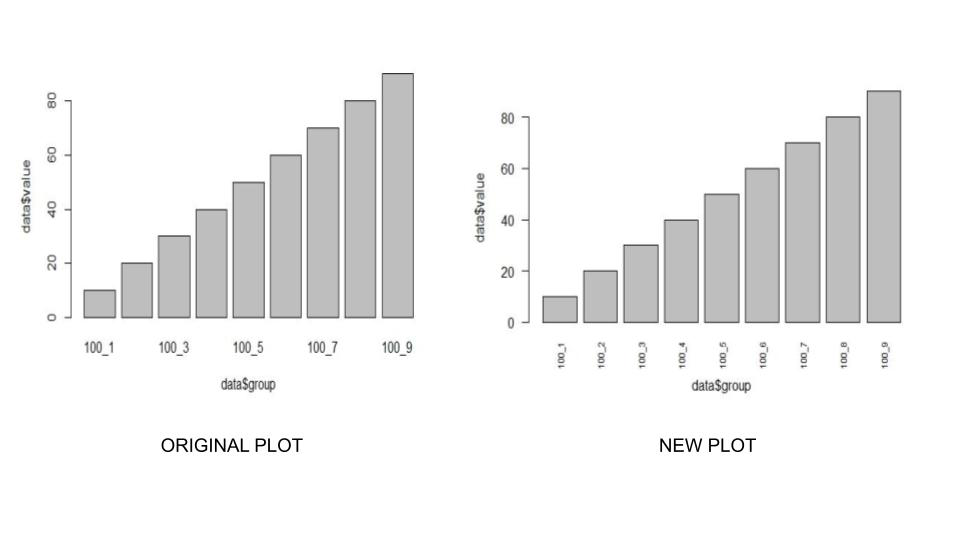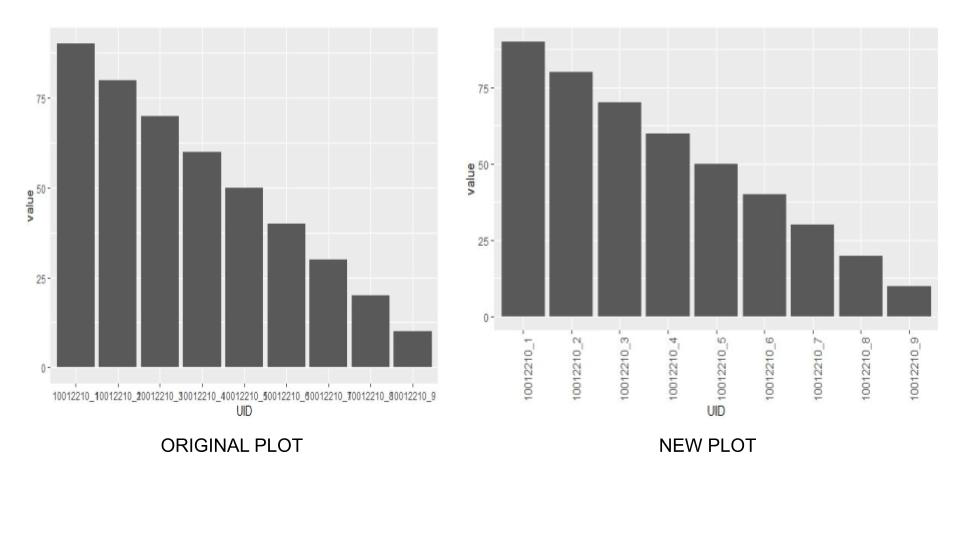# Display All X-Axis Labels of Barplot in R

• Last Updated : 09 May, 2021

While working on bar plots, there might be a situation where all the labels in the X-axis might not be visible due to the length of the variable names. This article deals with resolving the problem in the R programming language.

### Method 1: Using barplot()

In R language barplot() function is used to create a barplot. It takes the x and y-axis as required parameters and plots a barplot. To display all the labels, we need to rotate the axis, and we do it using the las parameter. To rotate the label perpendicular to the axis we set the value of las as 2, and for horizontal rotation, we set the value as 1. Secondly, to increase the font size of the labels we use cex.names to set the font size of the labels.

Syntax: barplot(data, xlab, ylab)

Parameter:

data is the data vector to be represented on y-axis
xlab is the label given to x-axis
ylab is the label given to y-axis

Example:

## R

 `rm``(list = ``ls``())`` ` `# Create the data``data <- ``data.frame``(value = ``c``(10,20,30,40,50,60,70,80,90),   ``                   ``group = ``paste0``(``"100_"``, 1:9))`` ` `# Original plot``barplot``(data\$value ~ data\$group)`` ` `# Modify x-axis labels``barplot``(data\$value ~ data\$group,     ``        ``las = 2,``        ``cex.names = 1)`

Output:### Method 2: Using ggplot

Another most popular library for drawing plots in R is ggplot2. We draw box plots using geom_bar() functions in ggplot2. To specify the changes are for the x-axis we use axis.text.x parameter in theme() function and use element_text() to specify the angle and font size.

Example:

## R

 `rm``(list = ``ls``())`` ` `# import library``library``(``"ggplot2"``)`` ` `# Create the data``data <- ``data.frame``(value = ``c``(90,80,70,60,50,40,30,20,10),   ``                   ``UID = ``paste0``(``"10012210_"``, 1:9))``head``(data)`` ` `# creating a bot plot``ggplot``(data, ``aes``(UID, value)) + ``  ``geom_bar``(stat = ``"identity"``) ``   ` ` ` `# ggplot2 plot with modified x-axis labels``ggplot``(data, ``aes``(UID, value)) +    ``  ``geom_bar``(stat = ``"identity"``) +``  ``theme``(axis.text.x = ``element_text``(angle = 90, size = 10))`

Output:My Personal Notes arrow_drop_up